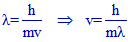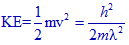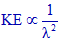# DE BROGLIE WAVELENGTH IIT JEE - NEET

## 1) The de Broglie wavelengths of electron waves in two orbits is 3:5. The ratio of kinetic energy of electrons will be:

#### (EAMCET 2009)

a) 25:9

b) 5:3

c) 9:25

d) 3:5

Logic:

The wavelength, λ of electron wave can be expressed by de Broglie's equation:It is is related to kinetic energy, KE as follows:i.e. KE is inversely proportional to λ2### Solution:

Hence the ratio of kinetic energies of electron will be:

KE1: KE2 =  λ22 : λ12 = 52 : 32 = 25 : 9

Conclusion:

The correct option is "a".

## Related questions

### 2) The velocities of two particles A and B are 0.05 and 0.02 m s-1 respectively. The mass of B is five times of mass of A. The ratio of their de Broglie’s wavelength is:

(EAMCET 2008-E)

a) 2:1

b) 1:4

c) 1:1

d) 4:1

Logic & solution:

From de Broglies equation, the ratio of wavelengths is related to their masses and velocities as follows:Conclusion:

Correct option is "a".

### 3) An electron is moving in Bohr's fourth orbit. Its de Broglie wavelength is λ . What is the circumference of the fourth orbit?

(EAMCET 2005-E)

1) 2/λ

b) 2λ

c) 4λ

d) -4/λ

Logic & solution:

According to de Broglies concept, the circumference of circle, 2πr must be equal to nλ.

Since n = 4 for fourth orbit,

Circumference, 2πr = 4λ

Conclusion:

Correct option is "c".

### 4) The de Broglie wavelength of a tennis balls of mass 60 g moving with a velocity of 10 m/s is approximately: ( Planck’s constant, h= 6.63x 10-34 J s)

(AIEEE 2003)

a) 10-33

b) 10-31

c) 10-16

d) 10-25 m

solution:

λ = h/mv = 6.63x 10-34 J s / 60 x 10-3 kg x 10 m s-1 = 1.1 x 10-33 m

Note: Use only SI units since Planck's constant is in SI units.

Conclusion:

Correct option is "c".

## Homework

1) What is the ratio of de Broglie's wavelengths of electron and proton moving with same velocities?

2) What is the wavelength of electron moving in 5th orbit of hydrogen atom?

Jump to Photo electric effect - Einstein - IIT JEE - NEET - IT JAM solved problems